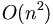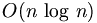Difference between revisions of "Fast Fourier Transform"

Fast Fourier Transform is an efficient algorithm for calculating the discrete fourier transform (DFT). Reduces the execution time by hundreds in some cases. Whereas DFT takes an order of$O(n^2)\,$ computations, FFT takes an order of$O(n\,\log\,n)$, and is definitely the preferred algorithm to used in all applications. The FFT in most implementations consistent of samples that are exactly a power of 2.